# How Focal Statistics works

Available with Spatial Analyst license.

Available with Image Analyst license.

The Focal Statistics tool performs a neighborhood operation that computes an output raster, where the value for each output cell is a function of the values of all the input cells that are in a specified neighborhood around that location. The function performed on the input is a statistic, such as the maximum, average, or sum of all values encountered in that neighborhood.

Conceptually, on execution, the algorithm visits each cell in the raster and calculates the specified statistic with the identified neighborhood. The cell for which the statistic is being calculated is referred to as the processing cell. The value of the processing cell, as well as all the cell values in the identified neighborhood, is included in the neighborhood statistics calculation.

The neighborhoods can overlap so that cells in one neighborhood may also be included in the neighborhood of another processing cell.

Example

To illustrate the neighborhood processing for Focal Statistics calculating a Sum statistic, consider the processing cell with a value of 5 in the following diagram. A rectangular 3 by 3 cell neighborhood shape is specified. The sum of the values of the neighboring cells (3 + 2 + 3 + 4 + 2 + 1 + 4 = 19) plus the value of the processing cell (5) equals 24 (19 + 5 = 24). So a value of 24 is given to the cell in the output raster in the same location as the processing cell in the input raster.

The above diagram demonstrates how the calculations are performed on a single cell in the input raster. In the following diagram, the results for all the input cells are shown. The cells highlighted in yellow identify the same processing cell and neighborhood as in the example above.

The shape of a neighborhood can be an annulus (a donut), a circle, a rectangle, or a wedge. The possible statistics that can be calculated within a neighborhood are mean, majority, maximum, median, minimum, minority, range, standard deviation, sum, and variety.

The Focal Statistics tool allows you to control the neighborhood type and statistic to be calculated.

## Neighborhood types

The shape of a neighborhood can be an annulus (a donut), a circle, a rectangle, or a wedge. Using a kernel file, you can also define a custom neighborhood shape, as well as assign different weights to specific cells in the neighborhood before the statistic is calculated.

Following are descriptions of the neighborhood shapes and how they are defined:

• Annulus
• The annulus shape is composed of two circles, one inside the other to make a donut shape. Cells with centers that fall outside the radius of the smaller circle but inside the radius of the larger circle will be included in processing the neighborhood. Therefore, the area that falls between the two circles constitutes the annulus neighborhood.
• The radius is identified in cells or map units, measured perpendicular to the x- or y-axis. When the radii are specified in map units, they are converted to radii in cell units. The resulting radii in cell units produce an area that most closely represents the area calculated using the original radii in map units. Any cell center encompassed by the annulus will be included in the processing of the neighborhood.
• The default annulus neighborhood is an inner radius of one cell and an outer radius of three cells.
• An example illustration of an annulus neighborhood follows: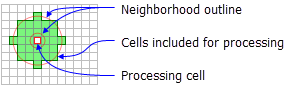Processing cell with the default annulus neighborhood example (inner radius 1 cell, outer radius 3 cells).

• Circle
• A circle neighborhood is created by specifying a radius value.
• The radius is identified in cell or map units, measured perpendicular to the x- or y-axis. When the radius is specified in map units, additional logic is used to determine which cells are included in the processing neighborhood. First, the exact area of a circle defined by the specified radius value is calculated. Next, the area is calculated for two additional circles, one with the specified radius value rounded down and one with the specified radius value rounded up. These two areas are compared to the result from the specified radius, and the radius of the area that is closest will be used in the operation.
• The default circle neighborhood radius is three cells.
• An example illustration of a circle neighborhood follows: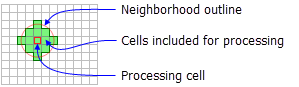Processing cell with a circle neighborhood example (radius = 2 cells).

• Rectangle
• The rectangle neighborhood is specified by providing a width and a height in either cells or map units.
• Only the cells with centers that fall within the defined object are processed as part of the rectangle neighborhood.
• The default rectangle neighborhood is a square with a height and width of three cells.
• The x,y position for the processing cell within the neighborhood, with respect to the upper left corner of the neighborhood, is determined by the following equations:

``````x = (width of the neighborhood + 1)/2
y = (height of the neighborhood + 1)/2``````

If the input number of cells is even, the x,y coordinates are computed using truncation. For example, in a 5 by 5 cell neighborhood, the x- and y-values are 3,3. In a 4 by 4 neighborhood, the x- and y-values are 2,2.

• Example illustrations of two rectangle neighborhoods follow: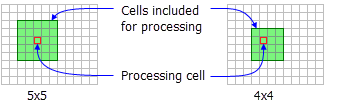Processing cell with two rectangle neighborhood examples.

• Wedge
• A wedge is a pie-shaped neighborhood specified by a radius, a starting angle, and an ending angle.
• The wedge extends counterclockwise from the starting angle to the ending angle. Angles are specified in arithmetic degrees from 0 to 360, where 0 is on the positive x-axis (3:00 on a clock), and can be integer or floating point. Negative angles can be used.
• The radius is identified in cells or map units, measured perpendicular to the x- or y-axis. When the radius is specified in map units, it is converted to a radius in cell units. The resulting radius in cell units produces an area that most closely represents the area calculated using the original radius in map units. Any cell center encompassed by the wedge will be included in the processing of the neighborhood.
• The default wedge neighborhood is from 0 to 90 degrees, with a radius of three cells.
• An example illustration of a wedge neighborhood follows: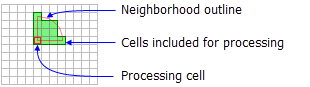Processing cell with the default wedge neighborhood example (radius 3 cells, start angle 0, end angle 90).

• Irregular
• Allows you to specify an irregularly shaped neighborhood around the processing cell.
• The irregular kernel file specifies which cell positions should be included within the neighborhood.
• The x,y position for the processing cell within the neighborhood, with respect to the upper left corner of the neighborhood, is determined by the following equations:

``````x = (width + 1)/2
y = (height + 1)/2``````

If the input number of cells is even, the x- and y-coordinates are computed using truncation.

• For the kernel file for an irregular neighborhood:

• The irregular kernel file is an ASCII text file that defines the values and shape of an irregular neighborhood. The file can be created with any text editor.
• The first line specifies the width and height of the neighborhood (the number of cells in the x direction, followed by a space, and the number of cells in the y direction).
• The subsequent lines give the values of each position in the neighborhood. The values are input in the same configuration as appears in the neighborhood they represent. A space between each value is necessary.
• The values in the kernel file should be either 0 (zero) or 1 (one). However, any value not equal to 0 will be interpreted as 1.
• A value of 0 (not a blank space) for a cell position indicates that the cell is not a member of the neighborhood and will not be used for processing. A value of 1 indicates that its corresponding cell (and value) is a member of the neighborhood.

• An example of an ASCII irregular kernel file and the neighborhood it represents follows: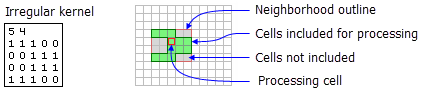Processing cell with an irregular neighborhood example.

• Weight
• Similar to the irregular neighborhood type, the weight neighborhood allows you to define an irregular neighborhood around the processing cell but also allows you to apply weights to the input values.
• The weight kernel file specifies which cell positions should be included within the neighborhood and the weights by which they will be multiplied.
• The weight neighborhood is only available for the mean, standard deviation, and sum statistics types.
• The x,y position for the processing cell within the neighborhood, with respect to the upper left corner of the neighborhood, is determined by the following equations:

``````x = (width + 1)/2
y = (height + 1)/2``````

If the input number of cells is even, the x- and y-coordinates are computed using truncation.

• For the kernel file for a weighted neighborhood:

• The weight kernel file is an ASCII text file that defines the values and shape of a weight neighborhood. The file can be created with any text editor.
• The first line specifies the width and height of the neighborhood (the number of cells in the x direction, followed by a space, and the number of cells in the y direction).
• The subsequent lines give the weight values of each position in the neighborhood. The values are input in the same configuration as appears in the neighborhood they represent. Positive, negative, and decimal values are all valid options to use as a weight. A space between each value is necessary.
• For locations in the neighborhood that are not to be part of the calculation, use a value of 0 at the corresponding location in the kernel file.

• An example of an ASCII-weighted kernel file and the neighborhood it represents follows: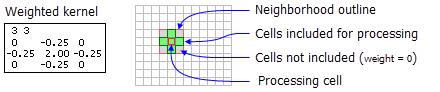Processing cell with a weighted neighborhood example.

## Statistics type

The available statistics are majority, maximum, mean, median, minimum, minority, range, standard deviation, and sum. The default statistics type is mean.

• Majority
• Only an integer raster can be used as input.
• The frequency of each unique cell value in a neighborhood is determined first. If there is a single value that has the highest frequency (is the most common), that value is returned as the output for that cell. However, there can be a tie, when there are two or more input values that have the highest frequency. In this case, the processing cell location will receive NoData in the output raster.
• Maximum
• If the input raster is integer, the values on the output raster will be integer; if the values on the input are floating point, the values on the output will be floating point.
• Mean
• The input can be an integer or a float raster.
• The output raster will always be floating point.
• The mean statistic can be used with the weight neighborhood type.
• Median
• The input can be an integer or a float raster.
• The output raster will always be floating point.
• If there is an odd number of valid cell values in the neighborhood, the median value is calculated by ranking the values and selecting the middle value. If there is an even number of values in a neighborhood, the values will be ranked and the middle two values will be averaged.
• Minimum
• If the input raster is integer, the values on the output raster will be integer; if the values on the input are floating point, the values on the output will be floating point.
• Minority
• Only an integer raster can be used as input.
• The frequency of each unique cell value in a neighborhood is determined first. If there is a single value that has the lowest frequency (is the least common), that value is returned as the output for that cell. However, there can be a tie, when there are two or more input values that have the lowest frequency. In this case, the processing cell location will receive NoData in the output raster.
• Percentile
• The input can be an integer or a float raster.
• The output raster will always be floating point.
• The result for the percentile statistic is calculated based on the following formula (Hyndman and Fan, 1996):
``pk = (k-1)/(n-1)``
• Range
• If the input raster is integer, the values on the output raster will be integer; if the values on the input are floating point, the values on the output will be floating point.
• The values for each cell location on the output raster are determined on a cell-by-cell basis by applying the formula:
``Focal Range = Focal Maximum – Focal Minimum``
• Standard deviation
• The output raster will always be floating point.
• The Standard deviation statistic can be used with the weight neighborhood type.
• Note that the standard deviation is calculated on the entire population (the N method); it is not estimated based on a sample (the N-1 method).
• Sum
• If the input raster is integer, the values on the output raster will be integer; if the values on the input are floating point, the values on the output will be floating point.
• Variety
• Only an integer raster can be used as input.

## Processing cells of NoData

The Ignore NoData in calculations option controls how NoData cells within the neighborhood window are handled. When this option is checked (the DATA option), any cells in the neighborhood that are NoData will be ignored in the calculation of the output cell value. When unchecked (the NODATA option), if any cell in the neighborhood is NoData, the output cell will be NoData.

If the processing cell is NoData, with the Ignore NoData option selected, the output value for the cell will be calculated based on the other cells in the neighborhood that have a valid value. If all of the cells in the neighborhood are NoData, the output will be NoData, regardless of the setting for this parameter.

## References

• Hyndman, R.J. and Fan, Y. (November 1996). "Sample Quantiles in Statistical Packages", The American Statistician 50 (4): pp. 361-365.